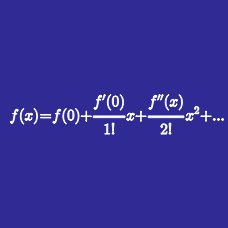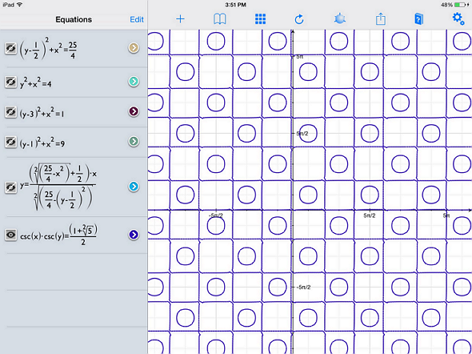Calculus

# Taylor Series: Level 4 ChallengesThe graph of $\csc(x)\csc(y)=\phi$ forms a bunch of squares and circles. The area of one of these circles is most nearly which of the following?

Remark: $\phi = \frac{1+\sqrt{5}}{2}$

Find the value of $S= \dfrac{\cos 0}{e^0 \cdot 0!}+ \dfrac{\cos 1}{e^1 \cdot 1!}+\dfrac{\cos 2}{e^2 \cdot 2!}+ \cdots$

$\textbf{Details and Assumptions}$

• In $\cos n$, $n$ is considered to be in $\textbf{radians}$.

Suppose a particle moves in a right-angled left spiral on an $xy$-grid. That is, it moves a distance $D_{1}(x)$ in a straight line, stops, makes a right-angled turn to it's "left", travels a distance $D_{2}(x)$ in a straight line, stops, makes a right angled turn to its "left", travels a distance $D_{3}(x)$ in a straight line and continues in this fashion forever.

If $D_{n}(x) = \dfrac{x^{n-1}}{(n-1)!}$ for $n \ge 1,$ and if $x = 2015,$ then find the magnitude of the straight line distance between the particle's starting and finishing points.

For all integers $n$, we define $\xi_n$ as follows: $\begin{cases} \xi_n = 1 & \text{if } n \equiv 0 \pmod{4} \text{ or } n \equiv 1 \pmod{4} \\ \xi_n= -1 & \text{if } n \equiv 2 \pmod{4} \text{ or } n \equiv 3 \pmod{4} \end{cases}$ For all $n \in \mathbb{Z^+}$, let $f(n)= \xi_0 \dbinom{n}{0} + \xi_1 \dbinom{n}{1} + \xi_2 \dbinom{n}{2} + \cdots + \xi_n \dbinom{n}{n}.$ Find $\left \lfloor 100 \left( \displaystyle \sum \limits_{n=0}^{\infty} \dfrac{f(n)}{n!} \right) \right \rfloor$.

Details and assumptions

• As an explicit example, since $4 \equiv 0 \pmod{4}$, $\xi_4= 1$, whereas $\xi_6 = -1$ since $6 \equiv 2 \pmod{4}$. Note that $\xi_0= \xi_1= 1$.

• The floor function $\lfloor x \rfloor$ denotes the largest integer less than or equal to $x$. For example, $\lfloor 3.25 \rfloor = 3, \lfloor 4 \rfloor= 4, \lfloor \pi \rfloor = 3$.

• You might use a scientific calculator for this problem.

If $\alpha$ and $\beta$ are roots of the equation $x^{2}-px+q=0$ then

$(\alpha+\beta)x-\frac{1}{2}(\alpha^{2}+\beta^{2})x^{2}+\frac{1}{3}(\alpha^{3}+\beta^{3})x^{3}- \ldots$ is equal to?

×